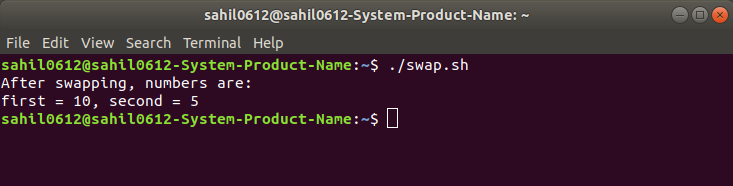GeeksforGeeks App
Open AppBrowser
Continue

# Bash shell script to swap two numbers

Given two numbers and the task is to swap them using the third variable.

Examples:

```Input: first = 2, second = 4
Output: first = 4, second = 2

Input: first = 5, second = 7
Output: first = 7, second = 5
```

Approach:

1. Store the value of the first number into a temp variable.
2. Store the value of the second number in the first number.
3. Store the value of temp into the second variable.

 `# !/bin/bash``  ` `# Program to swap two numbers``  ` `# Static input of the``# number``first=5``second=10``  ` `temp=\$first``first=\$second``second=\$temp`` ` `echo` `"After swapping, numbers are:"``echo` `"first = \$first, second = \$second"`

Output:

```After swapping, numbers are:
first = 10, second = 5
```

How to execute the bash files?

1. Write the bash code into a file and save that file with .sh extension i.e. filename.sh
2. Open terminal and execute the file using below command:
```./filename.sh
```My Personal Notes arrow_drop_up